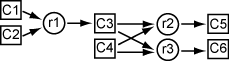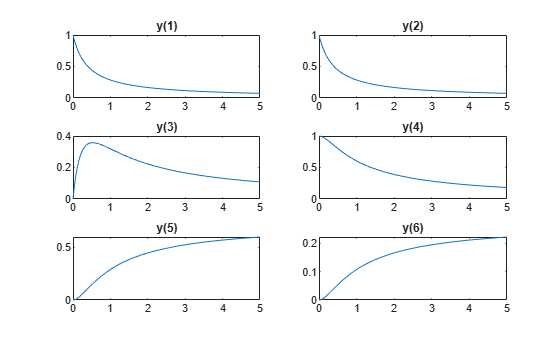# Fit ODE, Problem-Based

This example shows how to find parameters that optimize an ordinary differential equation (ODE) in the least-squares sense, using the problem-based approach.

### Problem

The problem is a multistep reaction model involving several substances, some of which react with each other to produce different substances.

For this problem, the true reaction rates are unknown. So, you need to observe the reactions and infer the rates. Assume that you can measure the substances for a set of times $t$. From these observations, fit the best set of reaction rates to the measurements.

### Model

The model has six substances, ${C}_{1}$ through ${C}_{6}$, that react as follows:

• One ${C}_{1}$ and one ${C}_{2}$ react to form one ${C}_{3}$ at rate ${r}_{1}$

• One ${C}_{3}$ and one ${C}_{4}$ react to form one ${C}_{5}$ at rate ${r}_{2}$

• One ${C}_{3}$ and one ${C}_{4}$ react to form one ${C}_{6}$ at rate ${r}_{3}$The reaction rate is proportional to the product of the quantities of the required substances. So, if ${y}_{i}$ represents the quantity of substance ${C}_{i}$, then the reaction rate to produce ${C}_{3}$ is ${r}_{1}{y}_{1}{y}_{2}$. Similarly, the reaction rate to produce ${C}_{5}$ is ${r}_{2}{y}_{3}{y}_{4}$, and the reaction rate to produce ${C}_{6}$ is ${r}_{3}{y}_{3}{y}_{4}$.

In other words, the differential equation controlling the evolution of the system is

`$\frac{\mathit{dy}}{\mathit{dt}}=\left[\begin{array}{c}-{\mathit{r}}_{1}{\mathit{y}}_{1}{\mathit{y}}_{2}\\ -{\mathit{r}}_{1}{\mathit{y}}_{1}{\mathit{y}}_{2}\\ -{\mathit{r}}_{2}{\mathit{y}}_{3}{\mathit{y}}_{4}+{\mathit{r}}_{1}{\mathit{y}}_{1}{\mathit{y}}_{2}-{\mathit{r}}_{3}{\mathit{y}}_{3}{\mathit{y}}_{4}\\ -{\mathit{r}}_{2}{\mathit{y}}_{3}{\mathit{y}}_{4}-{\mathit{r}}_{3}{\mathit{y}}_{3}{\mathit{y}}_{4}\\ {\mathit{r}}_{2}{\mathit{y}}_{3}{\mathit{y}}_{4}\\ {\mathit{r}}_{3}{\mathit{y}}_{3}{\mathit{y}}_{4}\end{array}\right].$`

Start the differential equation at time 0 at the point $y\left(0\right)=\left[1,1,0,1,0,0\right]$. These initial values ensure that all of the substances react completely, causing ${C}_{1}$ through ${C}_{4}$ to approach zero as time increases.

### Express Model in MATLAB

The `diffun` function implements the differential equations in a form ready for solution by `ode45`.

`type diffun`
```function dydt = diffun(~,y,r) dydt = zeros(6,1); s12 = y(1)*y(2); s34 = y(3)*y(4); dydt(1) = -r(1)*s12; dydt(2) = -r(1)*s12; dydt(3) = -r(2)*s34 + r(1)*s12 - r(3)*s34; dydt(4) = -r(2)*s34 - r(3)*s34; dydt(5) = r(2)*s34; dydt(6) = r(3)*s34; end ```

The true reaction rates are ${r}_{1}=2.5$, ${r}_{2}=1.2$, and ${r}_{3}=0.45$. Compute the evolution of the system for times zero through five by calling `ode45`.

```rtrue = [2.5 1.2 0.45]; y0 = [1 1 0 1 0 0]; tspan = linspace(0,5); soltrue = ode45(@(t,y)diffun(t,y,rtrue),tspan,y0); yvalstrue = deval(soltrue,tspan); for i = 1:6 subplot(3,2,i) plot(tspan,yvalstrue(i,:)) title(['y(',num2str(i),')']) end```### Optimization Problem

To prepare the problem for solution in the problem-based approach, create a three-element optimization variable `r` that has a lower bound of `0.1` and an upper bound of `10`.

`r = optimvar('r',3,"LowerBound",0.1,"UpperBound",10);`

The objective function for this problem is the sum of squares of the differences between the ODE solution with parameters `r` and the solution with the true parameters `yvals`. To express this objective function, first write a MATLAB function that computes the ODE solution using parameters `r`. This function is the `RtoODE` function.

`type RtoODE`
```function solpts = RtoODE(r,tspan,y0) sol = ode45(@(t,y)diffun(t,y,r),tspan,y0); solpts = deval(sol,tspan); end ```

To use `RtoODE` in an objective function, convert the function to an optimization expression by using `fcn2optimexpr`.

`myfcn = fcn2optimexpr(@RtoODE,r,tspan,y0);`

Express the objective function as the sum of squared differences between the ODE solution and the solution with true parameters.

`obj = sum(sum((myfcn - yvalstrue).^2));`

Create an optimization problem with the objective function `obj`.

`prob = optimproblem("Objective",obj);`

View the problem by calling `show`.

`show(prob)`
``` OptimizationProblem : Solve for: r minimize : sum(sum((RtoODE(r, extraParams{1}, extraParams{2}) - extraParams{3}).^2, 1)) extraParams variable bounds: 0.1 <= r(1) <= 10 0.1 <= r(2) <= 10 0.1 <= r(3) <= 10 ```

### Solve Problem

To find the best-fitting parameters `r`, give an initial guess `r0` for the solver and call `solve`.

```r0.r = [1 1 1]; [rsol,sumsq] = solve(prob,r0)```
```Solving problem using lsqnonlin. Local minimum found. Optimization completed because the size of the gradient is less than the value of the optimality tolerance. <stopping criteria details> ```
```rsol = struct with fields: r: [3×1 double] ```
```sumsq = 3.8669e-15 ```

The sum of squared differences is essentially zero, meaning the solver found parameters that cause the ODE solution to match the solution with true parameters. So, as expected, the solution contains the true parameters.

`disp(rsol.r)`
``` 2.5000 1.2000 0.4500 ```
`disp(rtrue)`
``` 2.5000 1.2000 0.4500 ```

### Limited Observations

Suppose that you cannot observe all the components of `y`, but only the final outputs `y(5)` and `y(6)`. Can you obtain the values of all the reaction rates based on this limited information?

To find out, modify the function `RtoODE` to return only the fifth and sixth ODE outputs. The modified ODE solver is in `RtoODE2`.

`type RtoODE2`
```function solpts = RtoODE2(r,tspan,y0) solpts = RtoODE(r,tspan,y0); solpts = solpts([5,6],:); % Just y(5) and y(6) end ```

The `RtoODE2` function simply calls `RtoODE` and then takes the final two rows of the output.

Create a new optimization expression from `RtoODE2` and the optimization variable `r`, the time span data `tspan`, and the initial point `y0`.

`myfcn2 = fcn2optimexpr(@RtoODE2,r,tspan,y0);`

Modify the comparison data to include outputs 5 and 6 only.

`yvals2 = yvalstrue([5,6],:);`

Create a new objective and new optimization problem from the optimization expression `myfcn2` and the comparison data `yvals2`.

```obj2 = sum(sum((myfcn2 - yvals2).^2)); prob2 = optimproblem("Objective",obj2);```

Solve the problem based on this limited set of observations.

`[rsol2,sumsq2] = solve(prob2,r0)`
```Solving problem using lsqnonlin. Local minimum possible. lsqnonlin stopped because the final change in the sum of squares relative to its initial value is less than the value of the function tolerance. <stopping criteria details> ```
```rsol2 = struct with fields: r: [3×1 double] ```
```sumsq2 = 2.1616e-05 ```

Once again, the returned sum of squares is essentially zero. Does this mean that the solver found the correct reaction rates?

`disp(rsol2.r)`
``` 1.7811 1.5730 0.5899 ```
`disp(rtrue)`
``` 2.5000 1.2000 0.4500 ```

No; in this case, the new rates are quite different from the true rates. However, a plot of the new ODE solution compared to the true values shows that `y(5)` and `y(6)` match the true values.

```figure plot(tspan,yvals2(1,:),'b-') hold on ss2 = RtoODE2(rsol2.r,tspan,y0); plot(tspan,ss2(1,:),'r--') plot(tspan,yvals2(2,:),'c-') plot(tspan,ss2(2,:),'m--') legend('True y(5)','New y(5)','True y(6)','New y(6)','Location','northwest') hold off```To identify the correct reaction rates for this problem, you must have data for more observations than `y(5)` and `y(6)`.

Plot all the components of the solution with the new parameters, and plot the solution with the true parameters.

```figure yvals2 = RtoODE(rsol2.r,tspan,y0); for i = 1:6 subplot(3,2,i) plot(tspan,yvalstrue(i,:),'b-',tspan,yvals2(i,:),'r--') legend('True','New','Location','best') title(['y(',num2str(i),')']) end```With the new parameters, substances ${C}_{1}$ and ${C}_{2}$ drain more slowly, and substance ${C}_{3}$ does not accumulate as much. But substances ${C}_{4}$, ${C}_{5}$, and ${C}_{6}$ have exactly the same evolution with both the new parameters and the true parameters.